# Bayes formula

(diff) ← Older revision | Latest revision (diff) | Newer revision → (diff)
A formula with which it is possible to compute a posteriori probabilities of events (or of hypotheses) from a priori probabilities. Let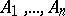be a complete group of incompatible events: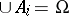,if. Then the a posteriori probability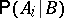of eventif given that eventwith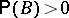has already occurred may be found by Bayes' formula: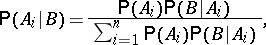(*)
where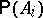is the a priori probability of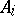,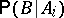is the conditional probability of eventoccurring given event(with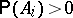) has taken place. The formula was demonstrated by T. Bayes in 1763.
Formula (*) is a special case of the following abstract variant of Bayes' formula. Letandbe random elements with values in measurable spaces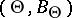andand let. Put, for any set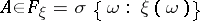,whereand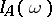is the indicator of the set. Then the measureis absolutely continuous with respect to the measure(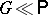) and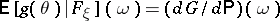, where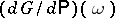is the Radon–Nikodým derivative ofwith respect to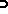.